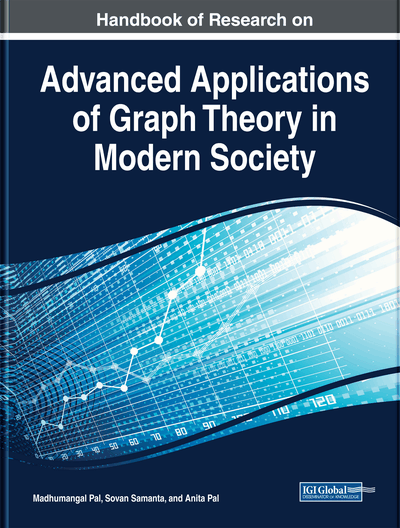# Sumset Valuations of Graphs and Their Applications

Sudev Naduvath, Germina K. Augusthy, Johan Kok
DOI: 10.4018/978-1-5225-9380-5.ch009
OnDemand:
(Individual Chapters)
Available
\$37.50
No Current Special Offers

## Abstract

Graph labelling is an assignment of labels to the vertices and/or edges of a graph with respect to certain restrictions and in accordance with certain predefined rules. The sumset of two non-empty sets A and B, denoted by A+B, is defined by A+B=\{a=b: a\inA, b\inB\}. Let X be a non-empty subset of the set \Z and \sP(X) be its power set. An \textit{sumset labelling} of a given graph G is an injective set-valued function f: V(G)\to\sP_0(X), which induces a function f+: E(G)\to\sP_0(X) defined by f+(uv)=f(u)+f(v), where f(u)+f(v) is the sumset of the set-labels of the vertices u and v. This chapter discusses different types of sumset labeling of graphs and their structural characterizations. The properties and characterizations of certain hypergraphs and signed graphs, which are induced by the sumset-labeling of given graphs, are also done in this chapter.
Chapter Preview
Top

## Introduction

In graph theory, graph labelling is an assignment of labels to the vertices and/or edges of a graph with respect to certain restrictions and in accordance with certain predefined rules. For the past few decades, graph labelling has become a fruitful research area in graph theory. Different graph labellings have resulted from practical considerations. They are not only of theoretical interests but have many practical implications also. For many applications, the edges or vertices of a given graph are given labels that are meaningful in the context.

### Graph Labelling

Valuation of a graphis a one to one mapping of the vertex set ofon to the set of all integers. Graph labeling has become a fertile research area in graph theory after the introduction of the notion of certain types number valuations, called-valuations, of graphs in Rosa (1967). The most popular one among the number valuations of graphs is-valuation or graceful labelling of a graph (see Golomb, 1972; Rosa, 1967) which is defined as an injective function

such that, when each edgeis assigned the label, the resulting edge labels are distinct. A graphis said to be edge-graceful if there exists a bijectionsuch that the induced mappinggiven bytaken over all edgesis a bijection.

Motivated by various problems in social networks, a set analogue of number valuations called set-valuation of graphs, has been introduced in Acharya (1983). In the number valuations, the elements of a graph are assigned to numbers while in the set-valuations, the elements of a graph are assigned to sets subject to certain conditions. The mathematical definition of a set-valuation of a graph concerned is as given below (see Acharya, 1983):

such that the functionis defined byfor every, whereis the set of all subsets ofandis the symmetric difference of sets. The definition can be generalised by allowing any binary operationof two sets in place of the symmetric differencein the above definitions.

Many significant and interesting studies on set-labelling of graphs have been done in the last two decades (see [1-10, 32, 33, 91]).

## Key Terms in this Chapter

Sequential Sumset Labeling: A sumset labeling with the collection of all vertex set-set-labels and edge-set-labels together with the nullset form the powerset of the ground set.

Weak Sumset Labeling: A sumset labeling in which the set-indexing number of each edge is equal to that of at least one of its end vertices.

Set-Indexing Number of an Element: The cardinality of the set-label of that element.

Set-Label Hypergraphs: The hypergraphs whose elements are the set-labels of the elements of a sumset graph.

Strong Sumset Labeling: A sumset labeling in which the set-indexing number of every edge is the product of the set-indexing numbers of its end vertices.

Sumset Signed Graph: A signed graph with a sumset labeling and whose signs are induced by the set-labels of its elements.

Graceful Sumset Labeling: A sumset labeling with the collection of all edge set-set-labels together with the null set and {0} form the power set of the ground set.

Sumset Labeling: An assignment of number sets to the elements of a graph such that the set-label of each edge is the sumset of the set-labels of its end vertices.

Arithmetic Sumset Labeling: A sumset labeling in which the set-labels of all vertices and edges of the graph are arithmetic progressions.

Topogenic Sumset Labeling: A sumset labeling with the collection of all vertex set-set-labels and edge-set-labels together with the nullset form a topology of the ground set.

## Complete Chapter List

Search this Book:
Reset